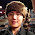Friday, 4 April 2014

Java (JPMML) Prediction using R PMML model (Part 2)

Last post showed how to generate a linear regression PMML model using R. This blog will load this PMML model into Java, via the excellent JPMML package, and perform predictions using a streaming test data set.

The method below represents how a PMML file is loaded in to memory using the JPMML library. Once the PMML has been load we are ready to perform prediction using streaming data. In this example our stream is a test csv file but in a production environment this can be real-time streams or some offline prediction mechanism using captured data ready for a start of day process.
```/**
* Load a PMML model from the file system.
*
* @param file
* @return PMML
* @throws Exception
*/
public final static PMML loadModel(final String file) throws Exception {

PMML pmml = null;

File inputFilePath = new File( file );

try( InputStream in = new FileInputStream( inputFilePath ) ){

Source source = ImportFilter.apply(new InputSource(in));
pmml = JAXBUtil.unmarshalPMML(source);

} catch( Exception e) {
logger.error( e.toString() );
throw e;
}
return pmml;
}
```

Now we have loaded the linear regression model as a PMML object we can start the real work of setting up the prediction processing. The next code section shows how to load, gain a predictive evaluator and get the list of input feature fields needed for the evaluator.

```// Load the file using our simple util function.
PMML pmml = JPMMLUtils.loadModel( pmmlFilePath );

// Now we need a prediction evaluator for the loaded model
PMMLManager mgr = new PMMLManager( pmml );

ModelEvaluator modelEvaluator = (ModelEvaluator) mgr.getModelManager(modelName, ModelEvaluatorFactory.getInstance());
Evaluator evaluator = modelEvaluator;

// Get the list of required feature set model needs to predict.
List requiredModelFeatures = evaluator.getActiveFields();
```

Below is the main body of the prediction routine. The code performs the following steps:

1. Parse the passed csv line into tokens and split into required variables.
2. Get the required feature variable field name.
3. Prepare the field to value mapping and assign to feature hashmap.
4. Perform the prediction (evaluate function).
5. Convert values back to original state and print out result.

``` // For each CSV line perform a predict.
while ((line = br.readLine()) != null) {

String[] tokens = line.split( cvsSplitBy );

double d =  Double.valueOf( tokens );
double e = Double.valueOf( tokens );

FieldName fieldName = requiredModelFeatures.get(0);

// In this instance I know there is only one feature
// For a production system this would be performed in a transformation stage and may collect data externally.
FieldValue value = evaluator.prepare(fieldName, Double.valueOf(d));
features.put( fieldName, value );

Map results = evaluator.evaluate( features );

// Convert back to original ring value so the prediction become meaningful.
double y = (Double)results.get( evaluator.getTargetField());
int predictedRings = (int) Math.abs( Math.pow( 10, y));

int expectedRings = (int) Math.abs( Math.pow( 10, e));

double diameter =  Math.pow( 10, d);
System.out.println(String.format("Diameter %f - Expected rings %d : Predicted rings: %d", diameter, expectedRings, predictedRings));
}
```

The section below presents the predictions performed using the JPMML linear regression evaluator using the passed offline R model. As you can see they are not perfect but the algorithm is generalizing reasonable well give the minor effort placed to build the model.
```    Diameter 0.225000 - Expected rings 7 : Predicted rings: 6
Diameter 0.530000 - Expected rings 11 : Predicted rings: 11
Diameter 0.500000 - Expected rings 11 : Predicted rings: 11
Diameter 0.460000 - Expected rings 8 : Predicted rings: 10
Diameter 0.270000 - Expected rings 7 : Predicted rings: 7
Diameter 0.510000 - Expected rings 11 : Predicted rings: 11
Diameter 0.295000 - Expected rings 10 : Predicted rings: 7
Diameter 0.450000 - Expected rings 9 : Predicted rings: 10
Diameter 0.350000 - Expected rings 7 : Predicted rings: 8
Diameter 0.195000 - Expected rings 6 : Predicted rings: 5
Diameter 0.435000 - Expected rings 18 : Predicted rings: 10
Diameter 0.410000 - Expected rings 11 : Predicted rings: 9
Diameter 0.455000 - Expected rings 9 : Predicted rings: 10
Diameter 0.440000 - Expected rings 11 : Predicted rings: 10
Diameter 0.270000 - Expected rings 7 : Predicted rings: 7
Diameter 0.495000 - Expected rings 11 : Predicted rings: 11
Diameter 0.525000 - Expected rings 11 : Predicted rings: 11
Diameter 0.205000 - Expected rings 3 : Predicted rings: 5
Diameter 0.510000 - Expected rings 10 : Predicted rings: 11
Diameter 0.375000 - Expected rings 8 : Predicted rings: 9
Diameter 0.135000 - Expected rings 5 : Predicted rings: 4
Diameter 0.465000 - Expected rings 11 : Predicted rings: 10
```

This now concludes the R to Java PMML integration sessions. My next algorithm I will be looking at is k-NN using the same technique. If you require any further information on how I did this please email me directly.

1.1.